# ISEE Middle Level Math : How to find the solution to an equation

## Example Questions

### Example Question #11 : Solve Real World And Mathematical Problems By Writing And Solving Equations: Ccss.Math.Content.6.Ee.B.7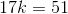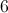Explanation:

Divide each side by: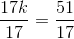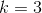### Example Question #61 : Equations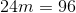Explanation:

Divide each side by: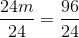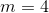### Example Question #11 : Solve Real World And Mathematical Problems By Writing And Solving Equations: Ccss.Math.Content.6.Ee.B.7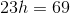Explanation:

Divide each side by: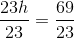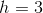### Example Question #14 : Solve Real World And Mathematical Problems By Writing And Solving Equations: Ccss.Math.Content.6.Ee.B.7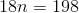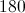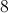Explanation:

Divide each side by: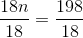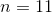### Example Question #11 : Solve Real World And Mathematical Problems By Writing And Solving Equations: Ccss.Math.Content.6.Ee.B.7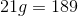What is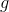?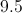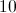Explanation:

Divide each side by: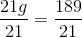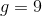### Example Question #2381 : Isee Middle Level (Grades 7 8) Mathematics Achievement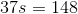What is?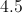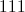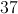Explanation:

Divide each side by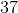: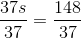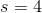### Example Question #62 : Equations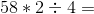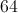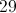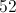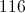Explanation:

First, following order of operations, multiply: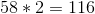Then, divide: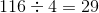### Example Question #61 : Equations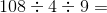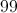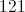Explanation:

First, following order of operations, divide: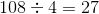Then, divide again: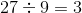### Example Question #11 : Solve Real World And Mathematical Problems By Writing And Solving Equations: Ccss.Math.Content.6.Ee.B.7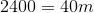What is?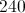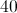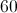Explanation:

Divide each side by: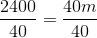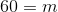### Example Question #12 : Solve Real World And Mathematical Problems By Writing And Solving Equations: Ccss.Math.Content.6.Ee.B.7

What is?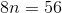Explanation:

Divide each side by: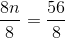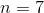### All ISEE Middle Level Math Resources